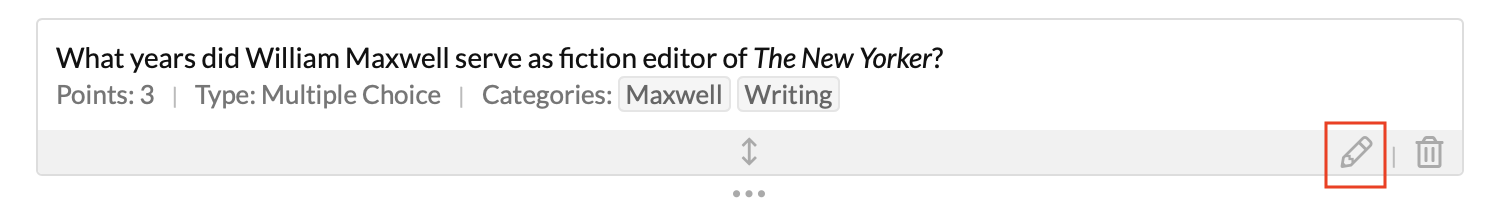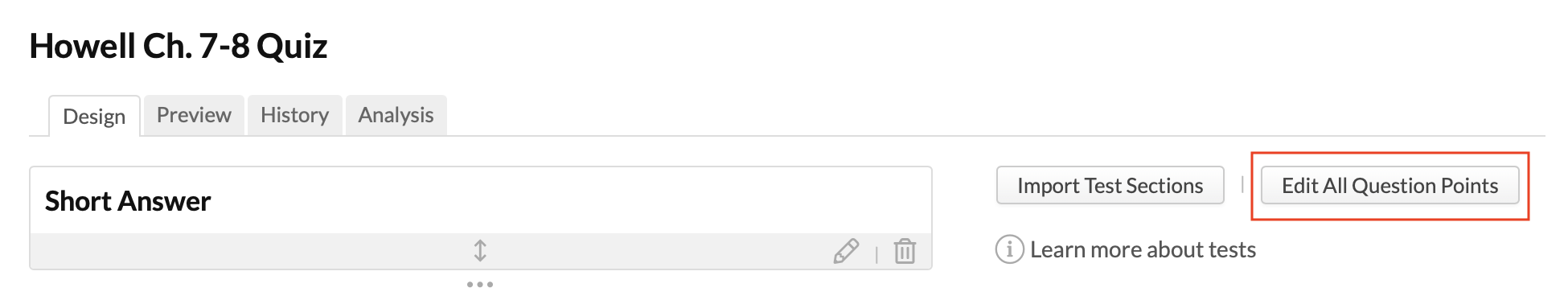Follow

# How to edit question points

After designing a test, you may find a need to adjust the question points. Here are all the ways you can do that.

### Individual questionsTo edit the points for an individual question:

1. Click   to edit the question.
2. Change the points.
3. Click Save.

If the test uses Fixed points calculation, you may end up with over- or under-assigned points. If so, fix it by editing the points on another question, changing the points calculation, or adjusting the test's points.

### All questions at onceYou can edit all the questions' points at once.

1. Click Edit all question points.
2. Adjust the points for the questions you'd like to change. You can also check to mark questions Extra Credit.
3. When you're done, click Save.

### Editing the test's pointsWhen you edit the test's points, you get several options for changing the test's points to fit the number of question points.

1. In the right column under the Information heading, click Points.
2. Depending on how the test is already set up, you'll have some or all of the following options:
• Adjust assignment points: Changes the assignment's points to match the total number of points you've given to your questions.
• Adjust question points: Automatically redistributes any over- or under-assigned points proportionally over all the test's questions.
• Change the Points calculation: This lets you change the calculation to one of the other two settings.
• Change the Points: This lets you enter a new number of points for the assignment.